# Camera Calibration and 3D Reconstruction¶

## gpu::StereoBM_GPU¶

class gpu::StereoBM_GPU

Class computing stereo correspondence (disparity map) using the block matching algorithm.

class StereoBM_GPU
{
public:
enum { BASIC_PRESET = 0, PREFILTER_XSOBEL = 1 };

enum { DEFAULT_NDISP = 64, DEFAULT_WINSZ = 19 };

StereoBM_GPU();
StereoBM_GPU(int preset, int ndisparities = DEFAULT_NDISP,
int winSize = DEFAULT_WINSZ);

void operator() (const GpuMat& left, const GpuMat& right,
GpuMat& disparity, Stream& stream = Stream::Null());

static bool checkIfGpuCallReasonable();

int preset;
int ndisp;
int winSize;

float avergeTexThreshold;

...
};


The class also performs pre- and post-filtering steps: Sobel pre-filtering (if PREFILTER_XSOBEL flag is set) and low textureness filtering (if averageTexThreshols > 0 ). If avergeTexThreshold = 0 , low textureness filtering is disabled. Otherwise, the disparity is set to 0 in each point (x, y) , where for the left image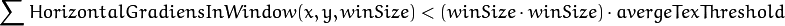This means that the input left image is low textured.

## gpu::StereoBM_GPU::StereoBM_GPU¶

Enables gpu::StereoBM_GPU constructors.

C++: gpu::StereoBM_GPU::StereoBM_GPU()
C++: gpu::StereoBM_GPU::StereoBM_GPU(int preset, int ndisparities=DEFAULT_NDISP, int winSize=DEFAULT_WINSZ)
Parameters: preset – Parameter presetting: BASIC_PRESET Basic mode without pre-processing. PREFILTER_XSOBEL Sobel pre-filtering mode. ndisparities – Number of disparities. It must be a multiple of 8 and less or equal to 256. winSize – Block size.

## gpu::StereoBM_GPU::operator ()¶

Enables the stereo correspondence operator that finds the disparity for the specified rectified stereo pair.

C++: void gpu::StereoBM_GPU::operator()(const GpuMat& left, const GpuMat& right, GpuMat& disparity, Stream& stream=Stream::Null())
Parameters: left – Left image. Only CV_8UC1 type is supported. right – Right image with the same size and the same type as the left one. disparity – Output disparity map. It is a CV_8UC1 image with the same size as the input images. stream – Stream for the asynchronous version.

## gpu::StereoBM_GPU::checkIfGpuCallReasonable¶

Uses a heuristic method to estimate whether the current GPU is faster than the CPU in this algorithm. It queries the currently active device.

C++: bool gpu::StereoBM_GPU::checkIfGpuCallReasonable()

## gpu::StereoBeliefPropagation¶

class gpu::StereoBeliefPropagation

Class computing stereo correspondence using the belief propagation algorithm.

class StereoBeliefPropagation
{
public:
enum { DEFAULT_NDISP  = 64 };
enum { DEFAULT_ITERS  = 5  };
enum { DEFAULT_LEVELS = 5  };

static void estimateRecommendedParams(int width, int height,
int& ndisp, int& iters, int& levels);

explicit StereoBeliefPropagation(int ndisp = DEFAULT_NDISP,
int iters  = DEFAULT_ITERS,
int levels = DEFAULT_LEVELS,
int msg_type = CV_32F);
StereoBeliefPropagation(int ndisp, int iters, int levels,
float max_data_term, float data_weight,
float max_disc_term, float disc_single_jump,
int msg_type = CV_32F);

void operator()(const GpuMat& left, const GpuMat& right,
GpuMat& disparity, Stream& stream = Stream::Null());
void operator()(const GpuMat& data, GpuMat& disparity, Stream& stream = Stream::Null());

int ndisp;

int iters;
int levels;

float max_data_term;
float data_weight;
float max_disc_term;
float disc_single_jump;

int msg_type;

...
};


The class implements algorithm described in [Felzenszwalb2006] . It can compute own data cost (using a truncated linear model) or use a user-provided data cost.

Note

StereoBeliefPropagation requires a lot of memory for message storage: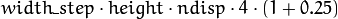and for data cost storage: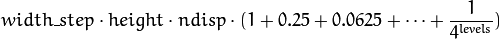width_step is the number of bytes in a line including padding.

## gpu::StereoBeliefPropagation::StereoBeliefPropagation¶

Enables the gpu::StereoBeliefPropagation constructors.

C++: gpu::StereoBeliefPropagation::StereoBeliefPropagation(int ndisp=DEFAULT_NDISP, int iters=DEFAULT_ITERS, int levels=DEFAULT_LEVELS, int msg_type=CV_32F)
C++: gpu::StereoBeliefPropagation::StereoBeliefPropagation(int ndisp, int iters, int levels, float max_data_term, float data_weight, float max_disc_term, float disc_single_jump, int msg_type=CV_32F)
Parameters: ndisp – Number of disparities. iters – Number of BP iterations on each level. levels – Number of levels. max_data_term – Threshold for data cost truncation. data_weight – Data weight. max_disc_term – Threshold for discontinuity truncation. disc_single_jump – Discontinuity single jump. msg_type – Type for messages. CV_16SC1 and CV_32FC1 types are supported.

StereoBeliefPropagation uses a truncated linear model for the data cost and discontinuity terms: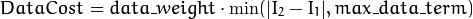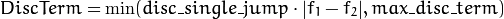For more details, see [Felzenszwalb2006].

By default, gpu::StereoBeliefPropagation uses floating-point arithmetics and the CV_32FC1 type for messages. But it can also use fixed-point arithmetics and the CV_16SC1 message type for better performance. To avoid an overflow in this case, the parameters must satisfy the following requirement: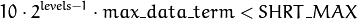## gpu::StereoBeliefPropagation::estimateRecommendedParams¶

Uses a heuristic method to compute the recommended parameters ( ndisp, iters and levels ) for the specified image size ( width and height ).

C++: void gpu::StereoBeliefPropagation::estimateRecommendedParams(int width, int height, int& ndisp, int& iters, int& levels)

## gpu::StereoBeliefPropagation::operator ()¶

Enables the stereo correspondence operator that finds the disparity for the specified rectified stereo pair or data cost.

C++: void gpu::StereoBeliefPropagation::operator()(const GpuMat& left, const GpuMat& right, GpuMat& disparity, Stream& stream=Stream::Null())
C++: void gpu::StereoBeliefPropagation::operator()(const GpuMat& data, GpuMat& disparity, Stream& stream=Stream::Null())
Parameters: left – Left image. CV_8UC1 , CV_8UC3 and CV_8UC4 types are supported. right – Right image with the same size and the same type as the left one. data – User-specified data cost, a matrix of msg_type type and Size(*ndisp, ) size. disparity – Output disparity map. If disparity is empty, the output type is CV_16SC1 . Otherwise, the type is retained. stream – Stream for the asynchronous version.

## gpu::StereoConstantSpaceBP¶

class gpu::StereoConstantSpaceBP

Class computing stereo correspondence using the constant space belief propagation algorithm.

class StereoConstantSpaceBP
{
public:
enum { DEFAULT_NDISP    = 128 };
enum { DEFAULT_ITERS    = 8   };
enum { DEFAULT_LEVELS   = 4   };
enum { DEFAULT_NR_PLANE = 4   };

static void estimateRecommendedParams(int width, int height,
int& ndisp, int& iters, int& levels, int& nr_plane);

explicit StereoConstantSpaceBP(int ndisp = DEFAULT_NDISP,
int iters    = DEFAULT_ITERS,
int levels   = DEFAULT_LEVELS,
int nr_plane = DEFAULT_NR_PLANE,
int msg_type = CV_32F);
StereoConstantSpaceBP(int ndisp, int iters, int levels, int nr_plane,
float max_data_term, float data_weight,
float max_disc_term, float disc_single_jump,
int min_disp_th = 0,
int msg_type = CV_32F);

void operator()(const GpuMat& left, const GpuMat& right,
GpuMat& disparity, Stream& stream = Stream::Null());

int ndisp;

int iters;
int levels;

int nr_plane;

float max_data_term;
float data_weight;
float max_disc_term;
float disc_single_jump;

int min_disp_th;

int msg_type;

bool use_local_init_data_cost;

...
};


The class implements algorithm described in [Yang2010]. StereoConstantSpaceBP supports both local minimum and global minimum data cost initialization algorithms. For more details, see the paper mentioned above. By default, a local algorithm is used. To enable a global algorithm, set use_local_init_data_cost to false .

## gpu::StereoConstantSpaceBP::StereoConstantSpaceBP¶

Enables the gpu::StereoConstantSpaceBP constructors.

C++: gpu::StereoConstantSpaceBP::StereoConstantSpaceBP(int ndisp=DEFAULT_NDISP, int iters=DEFAULT_ITERS, int levels=DEFAULT_LEVELS, int nr_plane=DEFAULT_NR_PLANE, int msg_type=CV_32F)
C++: StereoConstantSpaceBP::StereoConstantSpaceBP(int ndisp, int iters, int levels, int nr_plane, float max_data_term, float data_weight, float max_disc_term, float disc_single_jump, int min_disp_th=0, int msg_type=CV_32F)
Parameters: ndisp – Number of disparities. iters – Number of BP iterations on each level. levels – Number of levels. nr_plane – Number of disparity levels on the first level. max_data_term – Truncation of data cost. data_weight – Data weight. max_disc_term – Truncation of discontinuity. disc_single_jump – Discontinuity single jump. min_disp_th – Minimal disparity threshold. msg_type – Type for messages. CV_16SC1 and CV_32FC1 types are supported.

StereoConstantSpaceBP uses a truncated linear model for the data cost and discontinuity terms:For more details, see [Yang2010].

By default, StereoConstantSpaceBP uses floating-point arithmetics and the CV_32FC1 type for messages. But it can also use fixed-point arithmetics and the CV_16SC1 message type for better performance. To avoid an overflow in this case, the parameters must satisfy the following requirement:## gpu::StereoConstantSpaceBP::estimateRecommendedParams¶

Uses a heuristic method to compute parameters (ndisp, iters, levelsand nrplane) for the specified image size (widthand height).

C++: void gpu::StereoConstantSpaceBP::estimateRecommendedParams(int width, int height, int& ndisp, int& iters, int& levels, int& nr_plane)

## gpu::StereoConstantSpaceBP::operator ()¶

Enables the stereo correspondence operator that finds the disparity for the specified rectified stereo pair.

C++: void gpu::StereoConstantSpaceBP::operator()(const GpuMat& left, const GpuMat& right, GpuMat& disparity, Stream& stream=Stream::Null())
Parameters: left – Left image. CV_8UC1 , CV_8UC3 and CV_8UC4 types are supported. right – Right image with the same size and the same type as the left one. disparity – Output disparity map. If disparity is empty, the output type is CV_16SC1 . Otherwise, the output type is disparity.type() . stream – Stream for the asynchronous version.

## gpu::DisparityBilateralFilter¶

class gpu::DisparityBilateralFilter

Class refining a disparity map using joint bilateral filtering.

class CV_EXPORTS DisparityBilateralFilter
{
public:
enum { DEFAULT_NDISP  = 64 };
enum { DEFAULT_RADIUS = 3 };
enum { DEFAULT_ITERS  = 1 };

explicit DisparityBilateralFilter(int ndisp = DEFAULT_NDISP,

DisparityBilateralFilter(int ndisp, int radius, int iters,
float edge_threshold, float max_disc_threshold,
float sigma_range);

void operator()(const GpuMat& disparity, const GpuMat& image,
GpuMat& dst, Stream& stream = Stream::Null());

...
};


The class implements [Yang2010] algorithm.

## gpu::DisparityBilateralFilter::DisparityBilateralFilter¶

Enables the gpu::DisparityBilateralFilter constructors.

C++: gpu::DisparityBilateralFilter::DisparityBilateralFilter(int ndisp, int radius, int iters, float edge_threshold, float max_disc_threshold, float sigma_range)
Parameters: ndisp – Number of disparities. radius – Filter radius. iters – Number of iterations. edge_threshold – Threshold for edges. max_disc_threshold – Constant to reject outliers. sigma_range – Filter range.

## gpu::DisparityBilateralFilter::operator ()¶

Refines a disparity map using joint bilateral filtering.

C++: void gpu::DisparityBilateralFilter::operator()(const GpuMat& disparity, const GpuMat& image, GpuMat& dst, Stream& stream=Stream::Null())
Parameters: disparity – Input disparity map. CV_8UC1 and CV_16SC1 types are supported. image – Input image. CV_8UC1 and CV_8UC3 types are supported. dst – Destination disparity map. It has the same size and type as disparity . stream – Stream for the asynchronous version.

## gpu::drawColorDisp¶

Colors a disparity image.

C++: void gpu::drawColorDisp(const GpuMat& src_disp, GpuMat& dst_disp, int ndisp, Stream& stream=Stream::Null())
Parameters: src_disp – Source disparity image. CV_8UC1 and CV_16SC1 types are supported. dst_disp – Output disparity image. It has the same size as src_disp . The type is CV_8UC4 in BGRA format (alpha = 255). ndisp – Number of disparities. stream – Stream for the asynchronous version.

This function draws a colored disparity map by converting disparity values from [0..ndisp) interval first to HSV color space (where different disparity values correspond to different hues) and then converting the pixels to RGB for visualization.

## gpu::reprojectImageTo3D¶

Reprojects a disparity image to 3D space.

C++: void gpu::reprojectImageTo3D(const GpuMat& disp, GpuMat& xyzw, const Mat& Q, int dst_cn=4, Stream& stream=Stream::Null())
Parameters: disp – Input disparity image. CV_8U and CV_16S types are supported. xyzw – Output 3- or 4-channel floating-point image of the same size as disp . Each element of xyzw(x,y) contains 3D coordinates (x,y,z) or (x,y,z,1) of the point (x,y) , computed from the disparity map. Q –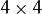perspective transformation matrix that can be obtained via stereoRectify() . dst_cn – The number of channels for output image. Can be 3 or 4. stream – Stream for the asynchronous version.

## gpu::solvePnPRansac¶

Finds the object pose from 3D-2D point correspondences.

C++: void gpu::solvePnPRansac(const Mat& object, const Mat& image, const Mat& camera_mat, const Mat& dist_coef, Mat& rvec, Mat& tvec, bool use_extrinsic_guess=false, int num_iters=100, float max_dist=8.0, int min_inlier_count=100, vector<int>* inliers=NULL)
Parameters: object – Single-row matrix of object points. image – Single-row matrix of image points. camera_mat – 3x3 matrix of intrinsic camera parameters. dist_coef – Distortion coefficients. See undistortPoints() for details. rvec – Output 3D rotation vector. tvec – Output 3D translation vector. use_extrinsic_guess – Flag to indicate that the function must use rvec and tvec as an initial transformation guess. It is not supported for now. num_iters – Maximum number of RANSAC iterations. max_dist – Euclidean distance threshold to detect whether point is inlier or not. min_inlier_count – Flag to indicate that the function must stop if greater or equal number of inliers is achieved. It is not supported for now. inliers – Output vector of inlier indices.